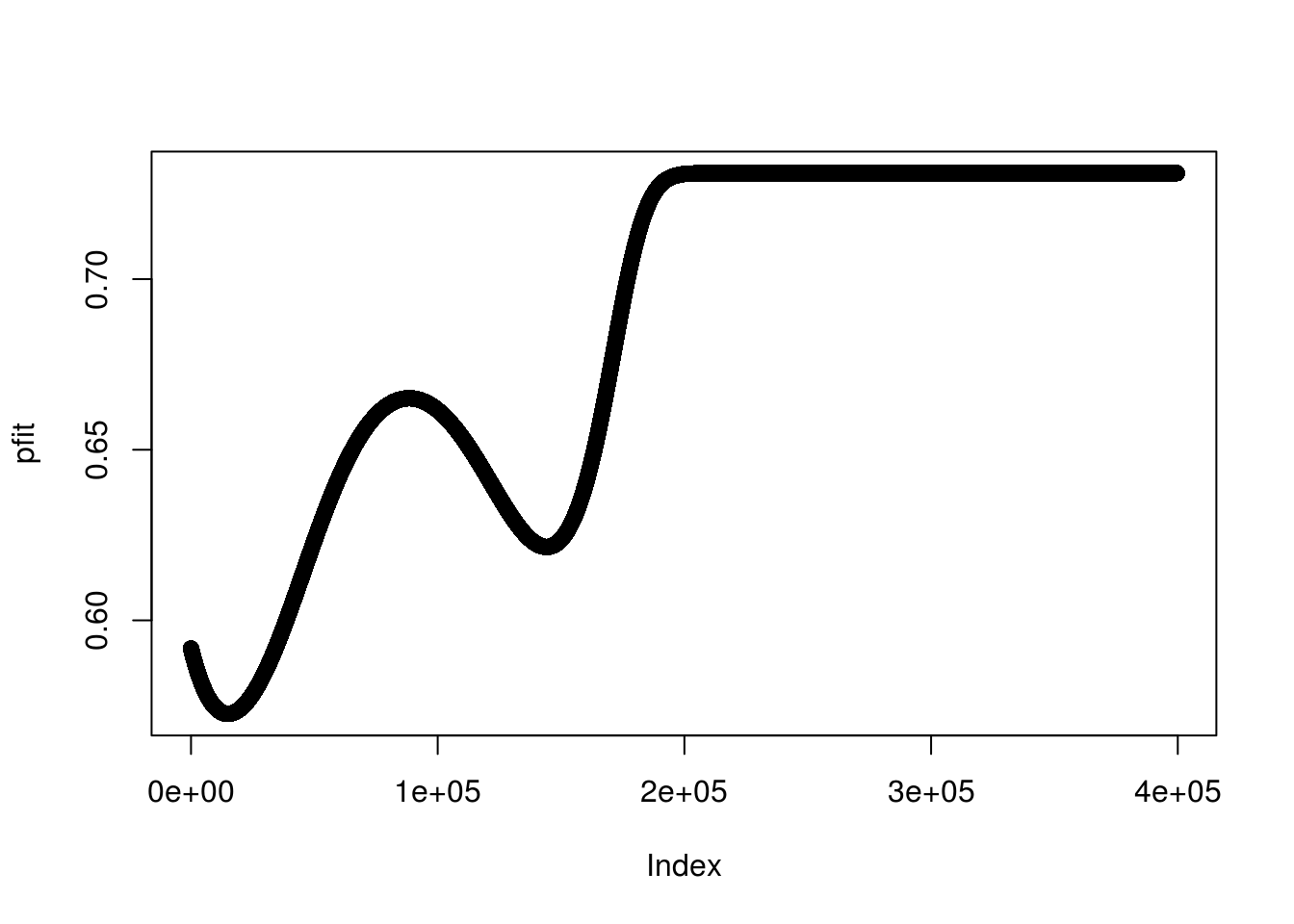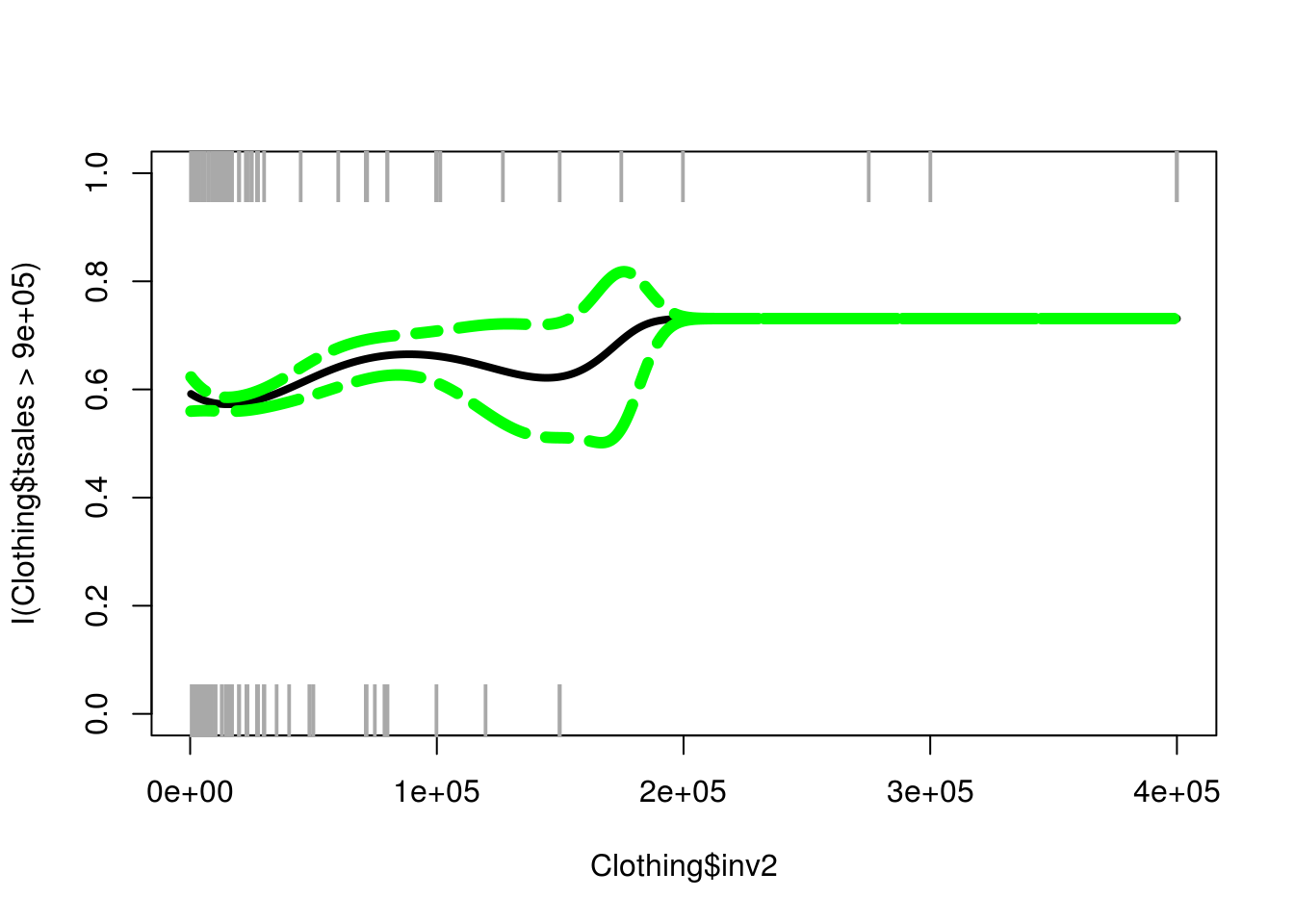# Logistic Polynomial Regression in R

Polynomial regression is used when you want to develop a regression model that is not linear. It is common to use this method when performing traditional least squares regression. However, it is also possible to use polynomial regression when the dependent variable is categorical. As such, in this post, we will go through an example of logistic polynomial regression.

Specifically, we will use the “Clothing” dataset from the “Ecdat” package. We will divide the “tsales” dependent variable into two categories to run the analysis. Below is the code to get started.

``library(Ecdat)``
``data(Clothing)``

There is little preparation for this example. Below is the code for the model

``fitglm<-glm(I(tsales>900000)~poly(inv2,4),data=Clothing,family = binomial)``

Here is what we did

1. We created an object called “fitglm” to save our results
2. We used the “glm” function to process the model
3. We used the “I” function. This told R to process the information inside the parentheses as is. As such, we did not have to make a new variable in which we split the “tsales” variable. Simply, if sales were greater than 900000 it was code 1 and 0 if less than this amount.
4. Next, we set the information for the independent variable. We used the “poly” function. Inside this function, we placed the “inv2” variable and the highest order polynomial we want to explore.
5. We set the data to “Clothing”
6. Lastly, we set the “family” argument to “binomial” which is needed for logistic regression

Below is the results

``summary(fitglm)``
``````##
## Call:
## glm(formula = I(tsales > 9e+05) ~ poly(inv2, 4), family = binomial,
##     data = Clothing)
##
## Deviance Residuals:
##     Min       1Q   Median       3Q      Max
## -1.5025  -0.8778  -0.8458   1.4534   1.5681
##
## Coefficients:
##                Estimate Std. Error z value Pr(>|z|)
## (Intercept)       3.074      2.685   1.145   0.2523
## poly(inv2, 4)1  641.710    459.327   1.397   0.1624
## poly(inv2, 4)2  585.975    421.723   1.389   0.1647
## poly(inv2, 4)3  259.700    178.081   1.458   0.1448
## poly(inv2, 4)4   73.425     44.206   1.661   0.0967 .
## ---
## Signif. codes:  0 '***' 0.001 '**' 0.01 '*' 0.05 '.' 0.1 ' ' 1
##
## (Dispersion parameter for binomial family taken to be 1)
##
##     Null deviance: 521.57  on 399  degrees of freedom
## Residual deviance: 493.51  on 395  degrees of freedom
## AIC: 503.51
##
## Number of Fisher Scoring iterations: 13``````

It appears that only the 4th-degree polynomial is significant and barely at that. We will now find the range of our independent variable “inv2” and make a grid from this information. Doing this will allow us to run our model using the full range of possible values for our independent variable.

``````inv2lims<-range(Clothing\$inv2)
inv2.grid<-seq(from=inv2lims,to=inv2lims)``````

The “inv2lims” object has two values. The lowest value in “inv2” and the highest value. These values serve as the highest and lowest values in our “inv2.grid” object. This means that we have values started at 350 and going to 400000 by 1 in a grid to be used as values for “inv2” in our prediction model. Below is our prediction model.

``predsglm<-predict(fitglm,newdata=list(inv2=inv2.grid),se=T,type="response")``

Next, we need to calculate the probabilities that a given value of “inv2” predicts a store has “tsales” greater than 900000. The equation is as follows.

``pfit<-exp(predsglm\$fit)/(1+exp(predsglm\$fit))``

Graphing this leads to interesting insights. Below is the code

``plot(pfit)``You can see the curves in the line from the polynomial expression. As it appears. As inv2 increase the probability increase until the values fall between 125000 and 200000. This is interesting, to say the least.

We now need to plot the actual model. First, we need to calculate the confidence intervals. This is done with the code below.

``````se.bandsglm.logit<-cbind(predsglm\$fit+2*predsglm\$se.fit,predsglm\$fit-2*predsglm\$se.fit)
se.bandsglm<-exp(se.bandsglm.logit)/(1+exp(se.bandsglm.logit))``````

The ’se.bandsglm” object contains the log odds of each example and the “se.bandsglm” has the probabilities. Now we plot the results

``````plot(Clothing\$inv2,I(Clothing\$tsales>900000),xlim=inv2lims,type='n')
points(jitter(Clothing\$inv2),I((Clothing\$tsales>900000)),cex=2,pch='|',col='darkgrey')
lines(inv2.grid,pfit,lwd=4)
matlines(inv2.grid,se.bandsglm,col="green",lty=6,lwd=6)``````In the code above we did the following.
1. We plotted our dependent and independent variables. However, we set the argument “type” to n which means nothing. This was done so we can add the information step-by-step.
2. We added the points. This was done using the “points” function. The “jitter” function just helps to spread the information out. The other arguments (cex, pch, col) our for aesthetics and our optional.
3. We add our logistic polynomial line based on our independent variable grid and the “pfit” object which has all of the predicted probabilities.
4. Last, we add the confidence intervals using the “matlines” function. Which includes the grid object as well as the “se.bandsglm” information.

You can see that these results are similar to when we only graphed the “pfit” information. However, we also add the confidence intervals. You can see the same dip around 125000-200000 were there is also a larger confidence interval. if you look at the plot you can see that there are fewer data points in this range which may be what is making the intervals wider.

Conclusion

Logistic polynomial regression allows the regression line to have more curves to it if it is necessary. This is useful for fitting data that is non-linear in nature.

## 1 thought on “Logistic Polynomial Regression in R”

1.Bendix Carstensen

The ci.pred() funktion from the Epi package does the confidence intervals for you. Incidentally for any link function you may use. The link function and the inverse link function is inside the glm object…